#Maths FridayWelcome to our #MathsFriday webpage

It will be updated as we cover new objectives and topics, so please visit regularly to keep up to date with 2L's maths news.Summer Term

Week 5

We have used these weeks to revisit objectives that were causing some confusion, inparticular tiem and money. We practised the following skills:

Time

Reading and converting analogue to digital.

Counting on a given time.

Knowing the position of: Quarter past, half past and quarter to on an analogue clock- knowing their digital equivalent.

Money

Using the fewest coins to make a given amount.

Using an alternative choice of coins to make the same amount.

Calculating change within £1.00, £5.00 and £10.00

Recognising value of coins and notes in order.

Week 3 & 4

We have revisited measures including:

cm and m

g and kg

ml and L

We calculated how many of the smaller unit in the larger.

Worked in half and quarter measures.

Recognised which unit of measure was the most appropriate for measuring in real life circumstances.

We found items that would be measured in eah given unit.

Week 1 & 2

We did it! National tests completed and we are feeling confident we did our best, that's what counts!

We spent the remainder of the week completing some fun maths activities in our seaside shop.

We worked out how much items would cost in total, and how much change we would get from £1.00, £5.00 and £10.00. We practised using different coins to make the same amounts.

We are all set to spend our pocket money on holiday now!Spring Term

Week 12

Monday 27th- Friday 31st March

This week we have revisited our multiplication and division facts:

We have used our 'switch- it' and 'inverse' facts to solve calculations and apply checking strategies.

3 x 5 = 15

5 x 3 = 15

15 = 3 x 5

15 = 5 x 3

15 divided by 3 = 5

15 divided by 5 = 3

Week 11

Monday 20th March- Friday 24th March

This week we have revisited our understanding of inverse operations, in particular addition and subtraction facts.

We used 'switch- it' facts as a checking strategy:

3 + 5 = 15      5 + 3 = 15

We also used inverse facts as another checking strategy:

15 - 5 = 10         15 - 10 = 5

We applied these to number sentences with 2 digit and 3 digit numbers, finding missing numbers.

245 + _____- = 1000

1000 - 245 = ________

We played lots of our favourite games to help us read and write larger numbers.

Week 10

This week we have been investigationg 3D shapes and their properties.

We have identified: vertices, faces and edges.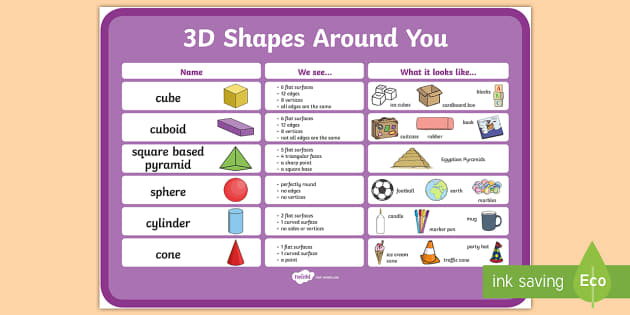We went on a hunt around the school to find 3D shapes in our environment!

Week 9

This week we have been applying our numeracy skills to problem solving and sequencing activities.

We have identified and applied patterns using shape, measures and number. We have identified missing numbers, predicted the next numbers in a sequence and identified rules for solving problems using these patterns.

Our reasoning skills have really helped us to complete these activities.

We have also used our addition and subtraction facts, and multiplication and division facts to solve more challenging problems, whilst practisig using our mathematical vocabualry.

Why not play some of these games to continue the learning at home?! Click the link below:Week 8

This week we have been investigating telling the time in analogue and digital.

We began recapping o'clock and half past, before reading the time in quarter past and quarter to, moving on to 5 minute intervals.

We practised drawing the hands on the clcok face, remembering that the hour hand is the shortest, the minute hand is the longest. Don't forget to use a ruler!

We read the digital clock firstly in 12 hours, moving on to 24 hours.

We watched a really helpful video that allowed us to make sense of telling the time, see below:

There are also links below to helpful games that can be played at home. Remember, they are not always accessible on a tablet or iPad.

Top Marks Games- Telling the TimeWeek 7

This week we have been investigating capacity: estimating, measuring and comparing.We used household bottles and containers such as water bottles and detergent bottles.

We then measured the capacity in ml and l by pouring the contents into a measuring jug or measuring cyclinder.

We also moved on to converting measures between ml and l:

1000ml = 1L

500ml = 0.5 L

250ml= 0.25 L

750 ml = 0.75 L

Why not continue the investigation at home using items in your recycle box?!

You could take a photograph and tweet us your hard work!

You could also play our 'Alien cookbook' game at home by clicking the link here.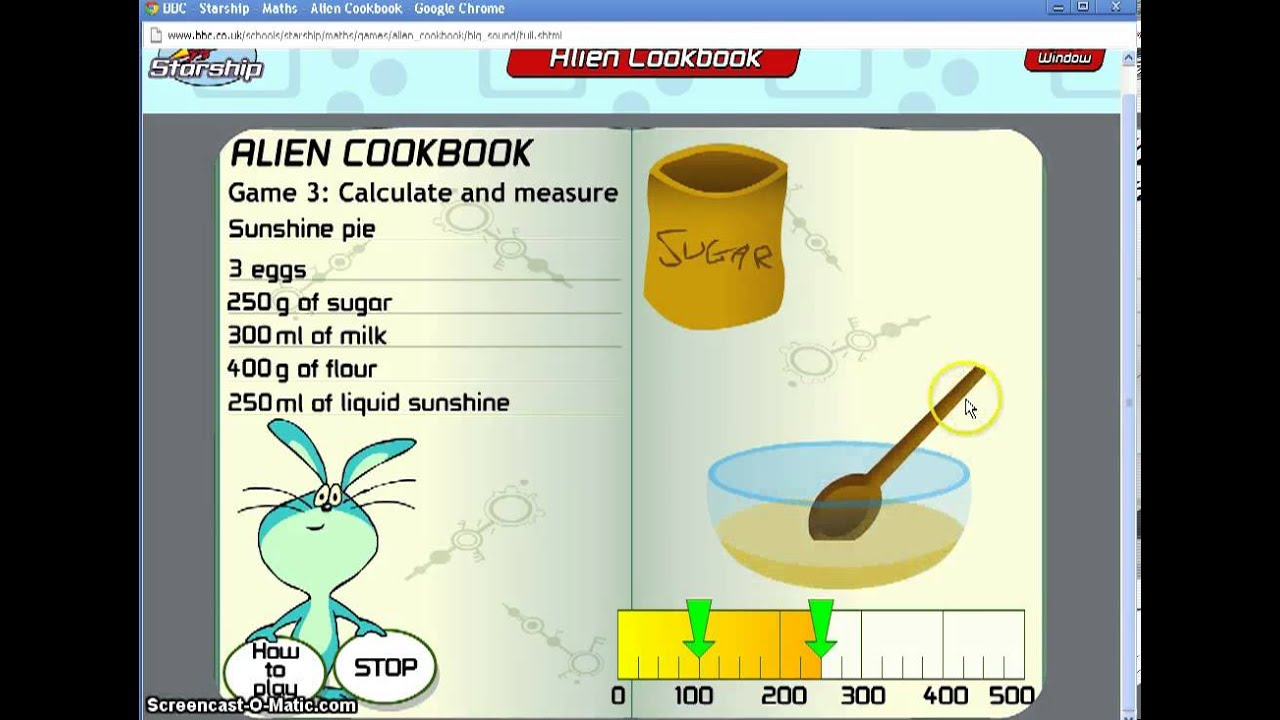Week 6

This week we have been investigating ways of doubling.

We have used partitioning: (Doubling diamonds)

Double 28

40        16

56

We have used halving facts to check our strategies:

2 x 28 = 56

56 / 2 = 28

28 & 28 = 56

We have played many games including 'Hit the Button' to check our facts.

We now feel really confident using our doubling facts!Week 5

This week we have been investigating fractions, in particular halves and quarters.

We have found fractions of shapes, food, numbers to 10 and in some cases, larger numbers.

We have used the vocabulary:whole, half, quarter, three quarters, to help us identify fractions of amounts.

We have also practised recognising equivalent fractions:

two quarters = one half

2/4 = 1/2

We learned that the number on the bottom is the denominator, which tells us how many parts altogether.

We learned that the numerator is the number on top, which tells us how many parts there are now, or shaded etc.

A great way of prsctising developing our understanding was using this helpful lego themed video!

Week 4

This week we have been investigating tally charts, bar charts and pictograms.

We used lots of fun online games to help us read, record and interpret information.

Click the links below to practise these at home.

We learned that tally charts are recorded using lines and a five bar gate, with totals recorded as a digit.

We learned that a bar graph has an x (horizontal) axis and a y (vertical axis). We practised reading the scale on each axis to make sure we could count in equal steps.

We also recorded results as a pictograph, recognising that one symbol could represent more than one item or object.

Week 3

This week we have been investigating the properties of 2D shapes: name, number of sides, number of corners and the number of lines of symmetry.

We have hunted shapes in the environment and made our own designs using 2D shapes, such as rockets, houses and cars.

We have enjoyed completing quizes online and playing interactive games. Our favourite website, Top Marks, has many links to fun games to play at home, reinforcing what we have been learning in school.

Click the link here to access shape games online.

We investigated shapes from 1 sided shapes to 10 sided shapes and practised drawing polygons with these number of sides.Week 2

Throughout week 2 we have been continuing to practise recognising, ordering and calculating amounts of mone; working with larger amounts, crossing over £1:00 and £10.00.

We have chosen the correct number of coins and notes to make given amounts, aswell as calculating change and the coins needed.See the notes below for guidance.

We have used the same fun online games to help us- see the links below.

Week 1

This week we have been practising identifying money; that is, recognising the value of coins and notes and making calculations based on these.

We have ordered coins from the smallest value to the largest, and extended to introduce notes too.

We have also added totals in a purse and calculated the amount needed to pay for an item, and change to be received.

We have used the counting on method: jumping to the nearest then next 10, to help us check our answers.

Click the images below to access the games we have played in class to help us with our learning this week.

Autumn Term

Week 12

This week we have been applying our number skills to reading and calculating measures.We learned that there are 1000g in 1 Kg and practised using our division skills to find out about other amounts, converting between g and kg.

E,g: 100g = 0.1 kg

200g = 0.2 kg

500g = 0.5 kg

750g = 0.75 kg

Once we were confident converting between measurements we practised balancing weighing scales by adding weights measured in both grams and kilograms.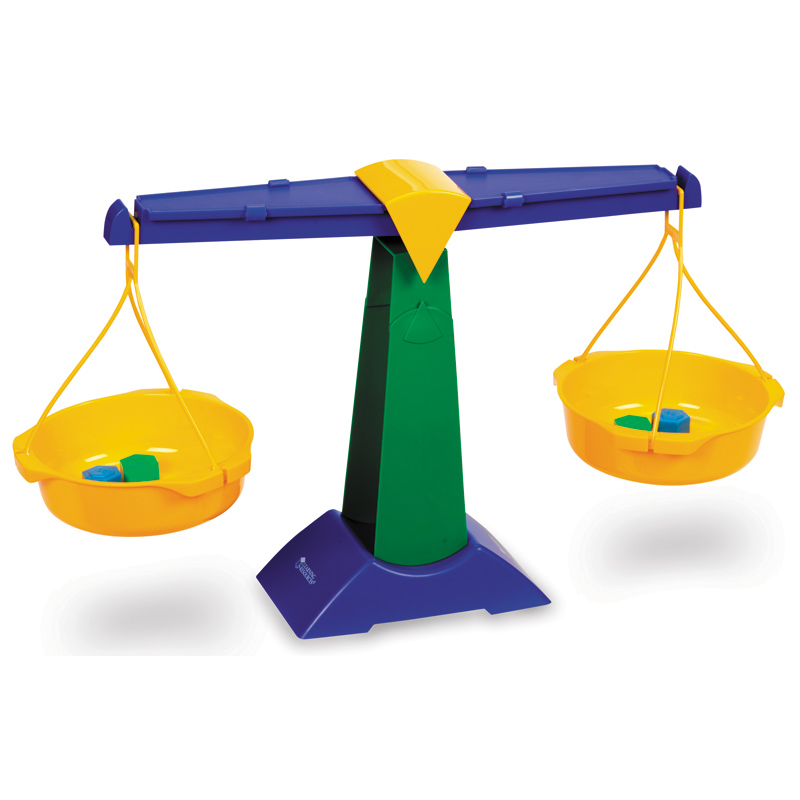E.g 5 kg = 3 kg + 2kg

We also added amounts using decimals:

4.5 kg = 2.5kg + 2 kg

We then read amounts and drew the pointer on weighing scales with differing scales.

E.g 0kg to 1 kg in units of 100gWe also estimated and compared the weight of a range of foods and objects. Once we were confident comparing weights, we made sure we were able to make estimates of weights for real life scenarios; such as, weighing ingredients, weight of a car, weight that could be held in a lift etc.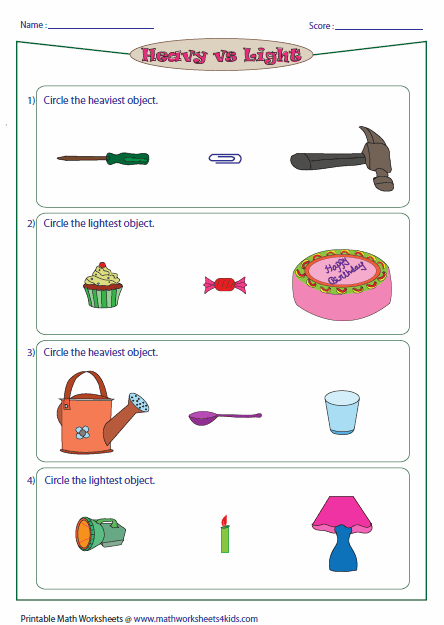A useful game to help us practise reading and ordering measures is linked below:

Washing line ordering- measuresWeek 11

This week we have been busy investigating ways to transform our outdoor learning area.There were so many fantastic ideas!

We used our number skills in everyday contexts including:

Measuring the perimeter of each area: L + L + W + W = P

We used measuring sticks, metre tapes and trundle wheels to measure in metres, to decimals to

one place e.g 6.6 metres.

We also calculated the area by multiplying L X W = A metre squared

We did this so that we would know what size fence and floor coverings to order.

We used the iPads to research ideas and cost materials. We recorded our ideas in a spreadsheet:

Item    Store     Cost per item    Cost for x number of itemsWe had lots of fun creating annotated drawings of our designs, explaining what we could make each area from, how it would be used and identifying the skills we coudl develop whilst using our designs.

We would love to bring these ideas to life and would really appreciate help to bring our ideas to fruition.

If you are able to offer your support in anyway we would love to hear from you!We will be holding a sponsored dance-a-thon in the coming weeks in the hope to raise funds for our project.Look out for letters in bags next week!

HomeworkPlease remember it is a great ideas to complete these tests in small chunks at a atime, sitting with children and discussing strategies. I know many children use a whiteboard as they do in class; this is a super ideas to practise strategies, a pad of paper will also be a super alternative.This half terms test focuses on multiplication and division, providinf opportunity for children to consolidate what they have learned in school.

Week 10

In our main maths lessons we continued practising our switch - it and inverse facts. We now feel really confident using a range of multiplication and division facts to solve problems!See Week 9 for a reminder of the methods and games used.

Guided ReasoningWe began our first Guided Reasoning Session this week.

These activities allow children to apply the numeracy skills acquired to problem solving activities, in everyday contexts.

Click here to access the 'Learning Wales Guide for Parents'. This is a useful booklet that fully explains the National Tests in a helpful guide.

This week we studied the time it would take to complete a journey based on a parade route.

We read the times given on the clock faces and explained the route taken based on the times given.

We then calculated how long it would take.

E.g First stop: 2:00pm

Last stop: 7:00pm

7:00pm -  2:00pm = 5hrs

The journey took 5 hrs from the school to the market.

Autumn Term Week 9

This week we have continued to rehearse doubling and halving strategies.

We have multiplied by 2 to double and divided by 2 to halve.

We linked these to our 'switch- it' facts:

3 x 2 = 6

2 x 3 = 6

We also linked these to inverse facts:

6 divided by 2 = 3

6 divided by 3 = 2

Once we were confident with these strategies, we applied them to our known number facts, working with numbers between 0 and 100.

E.g  5 x 6 = 30

6 x 5 = 30

30 divided by 6 = 5

30 divided by 5 = 6

We used blocks to help us practise our divisions if we needed to.At home, why not use beads or lego.We have played many games to apply the strategies we have gained, including our favourite 'Hit the button'- 'Doubles' and also introducing 'Halves'.

We linked our doubling and halving to our partitioning facts when working with 2 digit numbers:

Double 45 partitioned as

Double 40 = 80         Double 5 = 10

80 + 10 = 90

At times, we found it tricky to remeber to add 1 lot of 10 to our doubled ten, but we soon relaised the tens coloumn needed to change if the answer was greater than numbers 1-9.

We also used partitioning to halve 2 digit numbers:

Half 76 partitioned as

Half 70 = 35     Half 6 = 3

35 + 3

30 + 0 = 30       5 + 3 = 8

30 + 8 = 38

We intorduced repeated addition as a checking strategy, linking to our switch- it facts:

30 + 30 = 60     2 x 30 = 60    30 x 2 = 60

8 + 8 = 16      2 x 8 = 16     8 x 2 = 16

60 + 16 =

60 + 10 = 70      0 + 6 = 6

70 + 6 = 76

This sounds alot, but we were really confident using our number facts this week as we realised they can be linked so easily.

We just needed to recognise that we were working with a set of digits per question, then we were away!

We also continued practising counting in 4s during our warm-ups, to help us learn our multiplication facts.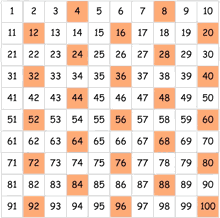Autumn Week 8

This week we are learning how to use our doubling and halving facts to solve problems. We have recognised that when doubling we multiply by 2, when halving, we divide by 2.

We are really confident doubling a number, but need to practise recording it as 'multiplied by 2' rather than a number add itself.

E.g Double 12 can be written as 12 + 12 = 24  or 12 x 2 = 24

Likewise, we need to practise recording halving:

Half 24 can be written as 24 - 12 = 12 or 24 divided by 2 = 12

We will be continuing to practise doubling and halving/ multiplying and dividing in week 9.

There are several great games we have been using to help us practise our doubling and halving, building on the partitioning work we have been doing this term.

Click on the images below to use them at home too.

Dartboard Doubles- Select your own level as you become more confidentHit the Button: Doubles- select your own level as you become more confidentRemember, if you find a great game, add it to our 'Maths Mat'!

This week we have also introduced our Four Times Tables as our 'Table of the Week'. We will continue to learn these in a variety of ways, including games, songs, quizes and drilling strategies.

The four times table test will take place on Friday18th November.

Homework this week is 'Four Times Tables'- see sheets in book bags on Friday.

Thank you to those of you who have started completing the online Alfie homework. There is no time limit in which to complete this, other than I would recommend completing before the next Alfie homework is issued at the end of this month.

A maths meeting for parents of children in Foundation Phase will be taking place on Thursday 10th November at 2:30pm with Mrs L Smith. See the main webpage and newsletter for further information.

Autumn Week 7

This week we have been continuing to identify place value, in numbers up to 100 and 1000.

We have identified a 3 digit number as having 'HTUs'- Hundreds, Tens and Units.

We learned that the column on the left becomes the next highest value, the hundreds.

We also learned that the column on the right always stays the same value, the units.

We ordered numbers according to their place value, using measures such as grams and kilograms.

We concentrated on identifying the place value and ordering of numbers with the same digit at the beginning.

E.g 234,  256, 298

We have again used the 'Washing Line' ordering game, which is a great tool for practising at home too.

We also used our partitioning facts to find a missing number. This was a little trickier as we sometimes forget that adding the units together made its' own number bond to 10.

E.g 25 + ______ = 100

5 + ____ = 10   (Answer 5)

20 + 10 = 30

30 + _____ = 100 (Answer 70)

70 + 5 = 75

Original sum complete:

25 + 75 = 100

#MathsFriday Homework information has been sent home and all information can be found on the Alfie drop down menu on the maths link.

Any questions, do not hesitate to ask.

Have a great half term!Autumn Week 6

We have again been learning how to partition 2- digit number this week. We have identified the tens and units in a 2-digit number.

As we gained confidence doing this, we began adding two, 2-digit numbers, with totals under 100.

We practised both mental recall and written methods.

We have been applying the rules we have learned throughout many different activities. (You may have seen our tweet!)

The method we have used is:

E.g 24 + 53 =

20 + 50 = 70           4 + 3 = 7

70 + 7 = 77

It is important children are recording the method, not just the answer, to truly assess the method being used is partitioning, not only counting on strategies, as this will give them the best foundation when it comes to completing increasingly challenging processes, such as adding HTUs.

A great resource we have used in class this week is 'Post Box partitioning'. Click the image below to access this great online game!Homework this week is in books bags today!

We have again practised our Two Times Table, and homework reinforces this, before our class test on Friday 21st October.

There is a drop down page on our #MathsFriday page, to the left, to help children learn their tables at home.

We are concentrating on the 2 Times Tables.

Autumn Week 5

We have been learning how to partition 2- digit numbers this week. We have identified the tens and units in a 2-digit number.

Once we were confident doing this, we began adding two, 2-digit numbers, with totals under 100.

We practised both mental recall and written methods. We have made a great start and will be continuing with this objective next week too, providing opportunity for children to apply what they have begun to understand.

The method we have used is:

E.g 24 + 53 =

20 + 50 = 70           4 + 3 = 7

70 + 7 = 77

A great resource we have used in class this week is 'Post Box partitioning'. Click the image below to access this great online game!Homework this week is in books bags today! We are introducing fortnightly multiplication tests to help children learn their multiplication facts. Look out for the note accompanying this weeks homework.

There is a drop down page on our #MathsFriday page, to the left, to help children learn their tables at home.

For the next fortnight we will be concentrating on the 2 Times Tables.

Autumn Week 4

We have been using a number line to help us count on and back, using the wording 'add' and 'subtract'.

We have been 'jumping' on to the next10, then adding the units that remain. That is, using our number bond facts.

E.g  14 + 9 = 23

14 + 6 = 20 + 3= 23

We have been illuastrating this on our lines.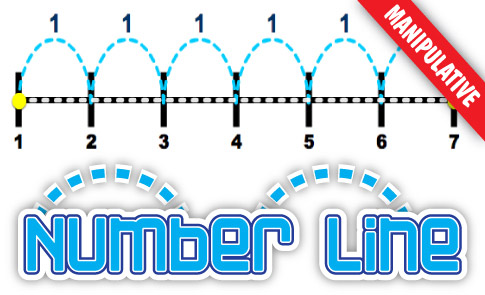Click the link here for useful number line games to play at home.***Homeowrk in bags- counting in multiples of 10 and 100 from any given number as we have been continuign to practise. ***

Autumn Week 3

We have been counting on and back in multiples of 2, 5, 10 or 100 to 100 and 1000.

Lots of confident responses when counting on in 5, e.g 0, 5, 10, 15, 20 and 10s  and 100s  e.g 2, 12, 24, 34. or 0, 100, 200, 300 etc.

Extra practise would be useful when counting from a number, not a common multiple e.g not in the multiplication tables e.g in 5s '3, 8, 13, 18'

In 10s,  e.g '13, 23, 33'

in 100s, e.g '137, 237, 337'

Homeowrk sheets have been put in book bages tonight.

Autumn Week 2

We have been counting on and back in multiples of 10 and 100, up to 1000.

Homework is in book bags today! 'Tens across the sea'

We have been learning that when you count to 100, in multiples of 10, the unit column stays the same, and the tens coloumn goes up by 1 digit, to the value of 10 each time.

We are beginning to apply this to counting in multiples of 100, to 1000.

We are learning that when counting in multiples in 100, the units and tens coloumn stay the stay, and the digits in the hundred go up by 1, to the value of 100.

Using the correct wording is helping us remember the rules.

We have used our 100 splat square and other usful games.

Autumn Week 1

We have been practising using a 100 square to count on in 2s, 5s and 10s to 100.

The children have really enjoyed the activities completed this week and have successfully met their objectives for the week.

**It would be helpful to continue practising number formation at home too please.**

Clcik on the images below to access the web pages we have used in class this week, either as teaching tolls, or games to introduce or consolidate methods used.

This week we have used:

Splat Square

Useful for revisitng number patterns up to 100, across any topic.Ordering numbersWe played ordering numbers up to 100